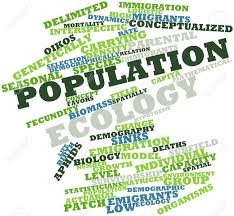Population Ecology
3 years ago
mrsbennettjms
Save
Edit
Host a game
Live GameLive
Homework
Solo Practice
Practice10 QuestionsShow answers
• Question 1
30 seconds
Q. What factor describes when populations increase because animals are moving into a population?
Emigration
Limiting Factors
Immigration
Exponential Growth
• Question 2
30 seconds
Q. What factor describes when populations decrease because animals are moving out of a population?
Emigration
Limiting Factors
Immigration
Exponential Growth
• Question 3
60 secondsQ. What does the graph represent?
Emigration
Exponential growth
Immigration
Logistic Growth
• Question 4
60 secondsQ. What does the graph represent?
Emigration
Exponential growth
Immigration
Logistic Growth
• Question 5
60 seconds
Q. Logistic growth has 3 phases. In Phase 1, the population grows rapidly. In Phase 2, population grows slowly. In Phase 3, growth stops. What causes growth to stop in the 3rd phase of logistic growth?
Emigration
Immigration
Exponential Growth
Carrying Capacity
• Question 6
30 seconds
Q. True or False: Limiting factors can be population dependent and population independent.
True/Cierto
False/Falso
• Question 7
60 seconds
Q. Which limiting factor would increase due to the number of individuals in a population?
Unusual weather
Natural disaster
Births
Disease
• Question 8
60 seconds
Q. Which limiting factor is independent of the number of individuals in a population?
competition
parasitism/disease
stress
natural disasters
• Question 9
60 seconds
Q. Human population grew exponentially after the industrial revolution. Which of the following DOES NOT help determine patterns in human population growth?
age structure
births
deaths
limiting factors
• Question 10
30 seconds
Q. Which of the following is TRUE of predator/prey relationships?